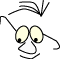Mathematics>Algebra

# A few algebraic topics

What
Some basic concepts like vector and matrix

In computer graphics it is crucial that we have knowledge about some basic mathematics. In this module we will, as in the other mathematics modules, go straight to the point and treat the mathematics as a tool. We will not worry about proof or generalization, but focus on the practical issues.

The advantage is that we quickly can establish the apparatus we need. The disadvantage is that we may lose the general concepts that could be necessary when we need to study the mathematics more deeply.

## Matrices

We know that we can add two matrices, or two vectors, if the number of rows and columns are equal in both.

A=B+C
aij=bij+cij

all rows i and columns j

### Multiplication

We know that we can multiply two matrices if the number of columns in the first matrix equals the number of rows in the second (n). The result matrix gets the same number of rows, nr, as the first matrix and the same number of columns, nk, as the second one.

A=B*C

for(r=1;r<=nr; r++)
for(k=1;k<=nk; k++)
{
ar,k=sum(br,i*ci,k)
i=1..n
}

### Transposition

We can transpose matrices. To transpose a matrix means technically to mirror it around the diagonal. The diagonal is the elements that have 2 equal indexes. We write MT for the transposed matrix M.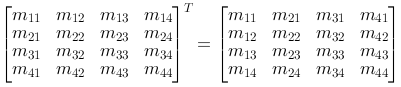The transposed for a row vector P is a corresponding column vector.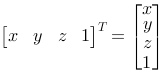Remember the modules about transformations in the plane and the space. By inspection we can see that the matrices we use in the expressions P2=M·P1 are the transposed of the ones we use in P2=P1·M.

### Inverting

Matrix B is said to be the inverted of matrix A if A·B=I, the identity matrix. Geometrically we perceive this so that a matrix is the inverted of another if it neutralizes a transformation, that means, does the opposite. In some cases we can by reasoning find the inverted of a matrix by using geometrical reasoning. For example we can assume that the inverse matrix for a rotation around the z-axis with the angle v is a matrix with the rotation -v.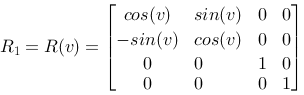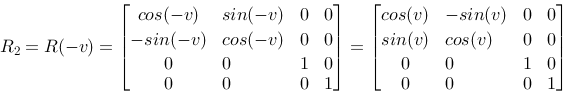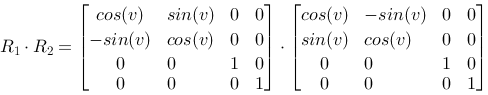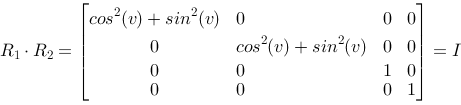since

cos2(v)+sin2(v)=1

for all v.

If we give up geometrical knowledge about the matrices we have to use standard algorithms to find the inverted of a matrix. We'll not discuss this here, but algorithms like these can be found in most numerical libraries.

Graphical packages sometimes have use for operating with inverted transformations. A case could be when we want to interactively point with the mouse on a part of a model and wish to find what object we pointed at. If we assume that the model has been transformed we should be able to transform the mouse point back into the model by the inverse transformation.

In the MS-Windows API the routines LPtoDP (Logical Point to Device Point) and DPtoLP (Device Point to Logical Point) handles transformations between model coordinates and screen coordinates. Here are both model and screen (device) coordinates given in the plane.

We will see in the module: To identify by pointing, that OpenGL uses another strategy to identify objects that are pointed at in a three dimensional model.

## Vectors

### The length of a vector

Assume a vector A. The length of A in n-space is defined to be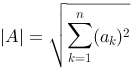This is a generalization of Pythagoras. For n=3, the space, we can illustrate it like this: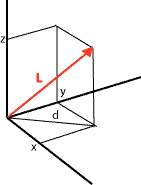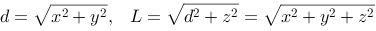### Scalar product

The scalar product of two vectors is a number, a scalar. Assume two vectors A and B. The scalar product is defined as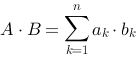where n is the dimension of the vectors.

We set n=3 and describe the vectors A and B as row vectors: A=(2,3,5) and B=(1,-6,2). The scalar product becomes: A·B=2·1-3·6+5·2=-6

Further we see that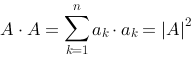We can for example let A be described by (x,y,z), and we can write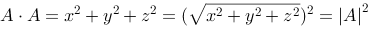We will use the scalar product to deduce a practical way to find the angle between to vectors. Consider the two vectors A and B.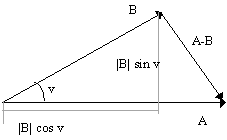We can write:

|A-B|2 =(|A|-|B|cos(v))2+|B|2sin2(v)
=|A|2+|B|2cos2(v)-2|A||B|cos(v)+|B|2sin2(v)
=|A|2+|B|2(cos2(v)+sin2(v))-2|A||B|cos(v)
=|A|2+|B|2-2|A||B|cos(v)

We can also write:

|A-B|2
=(A-B)(A-B)
=AA-2AB+BB
=|A|2+|B|2-2AB

Together this gives us:

|A|2+|B|2-2AB=|A|2+|B|22|A||B|cos(v)
AB=|A||B|cos(v)

This is interesting and useful because it tells us that if we know two vectors, we can find the cosine of the angle between them.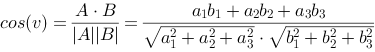and if the vectors are normalized, their length equal 1, we can write.

cos(v)=AB.

In OpenGL the angle between different directions (vectors) is used, among other things to calculate reflected light. To make OpenGL act reasonable, we usually have to state the normal vectors for surfaces we specify:

`  glNormal3f(x,y,z)`

Internally OpenGL would like to do calculations on these as normalized vectors. We can avoid the work with normalizing vectors ourselves by turning on automatic normalization:

```  glEnable(GL_NORMALIZE)
```

### The Cross product

The cross product of two vectors is a new vector, AxB=C. The vector C is placed in a 90 degrees angle to A and B and its direction is in a way so that the vectors A, B and C creates a right hand system. C is the normal of the plane spawned by A and B.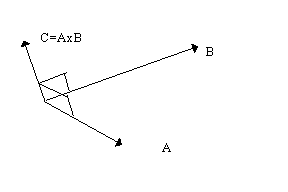The cross product of two vectors in space is defined as:

C=AxB=(a2b3-a3b2, a3b1-a1b3, a1b2-a2b1)

This is useful in situations where we are specifying normals for surfaces. We know that if we know three points (not in line) in a plane we can calculate the plane's normal.

References

This material is described in most books on computer graphics.